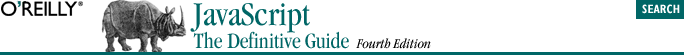home | O'Reilly's CD bookshelfs | FreeBSD | Linux | Cisco | Cisco Exam## 11.2. By Value Versus by Reference

There are two fundamentally distinct ways to manipulate data values. These techniques are called "by value" and "by reference." When a value is manipulated by value, it is the value of the datum that matters. In an assignment, a copy of the actual value is made and that copy is stored in a variable, object property, or array element; the copy and the original are two totally independent values that are stored separately. When a datum is passed by value to a function, a copy of the datum is passed to the function; if the function modifies the value, the change affects only the function's copy of the datum -- it does not affect the original datum. Finally, when a datum is compared by value to another datum, the two distinct pieces of data must represent exactly the same value (which usually means that a byte-by-byte comparison finds them to be equal).

The other way of manipulating a value is by reference. With this technique, there is only one actual copy of the value; references to that value are manipulated. If a value is manipulated by reference, variables do not hold that value directly; they hold only references to it. It is these references that are copied, passed, and compared. So, in an assignment made by reference, it is the reference to the value that is assigned, not a copy of the value and not the value itself. After the assignment, the new variable refers to the same value that the original variable refers to. Both references are equally valid and both can be used to manipulate the value -- if the value is changed through one reference, that change also appears through the original reference. The situation is similar when a value is passed to a function by reference. A reference to the value is passed to the function, and the function can use that reference to modify the value itself; any such modifications are visible outside the function. Finally, when a value is compared to another by reference, the two references are compared to see if they refer to the same unique copy of a value; references to two distinct values that happen to be equivalent (i.e., consist of the same bytes) are not treated as equal.

These are two very different ways of manipulating values, and they have important implications that you should understand. Table 11-2 summarizes these implications. This discussion of manipulating data by value and by reference has been a general one, but the distinctions apply to all programming languages. The sections that follow explain how these distinctions apply specifically to JavaScript; they discuss which data types are manipulated by value and which are manipulated by reference.

#### Table 11-2. By value versus by reference

By value

By reference

Copy

The value is actually copied; there are two distinct, independent copies.

Only a reference to the value is copied. If the value is modified through the new reference, that change is also visible through the original reference.

Pass

A distinct copy of the value is passed to the function; changes to it have no effect outside the function.

A reference to the value is passed to the function. If the function modifies the value through the passed reference, the modification is visible outside the function.

Two distinct values are compared (often byte by byte) to see if they are the same value.

Two references are compared to see if they refer to the same value. Two references to distinct values are not equal, even if the two values consist of the same bytes.